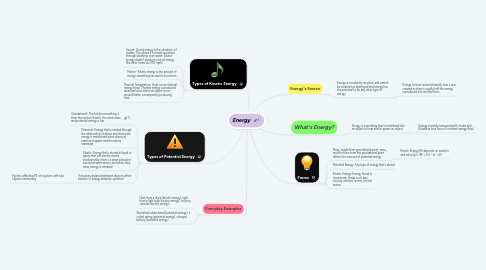# EnergyKalyn THOMPSON
Get Started. It's FreeEnergy## 1. Types of Potential Energy

### 1.4. How does distance between objects affect transfer of energy between systems?

1.4.1. Factors affecting PE of a system with two objects interacting:

## 4. What's Energy?

### 4.1. Energy is something that's transferred into an object to heat and/or power an object

4.1.1. Energy is mainly measured with Joules and Kilowatts (one hour of constant energy flow)

## 5. Energy's Source

### 5.1. Energy is constantly recycled, and cannot be created nor destroyed and energy has the potential to be any other type of energy

5.1.1. Energy is never restored exactly how it was created as there's usually half the energy reproduced into another form

## 6. Forms

### 6.1. Mass, height from gravitational point, mass, and the force from the gravitational point affects the amount of potential energy

6.1.1. Kinetic Energy/KE depends on mass/m and velocity/v (KE = 0.5 • m • v2)